American Journal of Civil Engineering
Volume 4, Issue 2, March 2016, Pages: 61-67

The Research of High Slope Instability Mechanism Based on Slide

Peng Qu1, 2, *, Cong Li2, Xueming Jia2

1College of Civil Engineering, Chongqing Jiaotong University, Chongqing, China

2China Merchants Chongqing Communications Technology Research & Design Institute Co., Ltd., Chongqing, China(Peng Qu)

*Corresponding author

Peng Qu, Cong Li, Xueming Jia. The Research of High Slope Instability Mechanism Based on Slide. American Journal of Civil Engineering. Vol. 4, No. 2, 2016, pp. 61-67. doi: 10.11648/j.ajce.20160402.14

Received: March 14, 2016; Accepted: April 5, 2016; Published: April 11, 2016

Abstract: In the highway engineering, as lacking cognition of mechanism in high slope stability caused landslide and collapse, which brought great damage to highway operation. The high slope protection and disaster management are given more and more attention with the continuous development of highway construction. This paper focus on slope stability analysis, the application ofanchorage is suitable to disaster prevention and control in high slope. The Slide software is adopted to simulatea high slope in Chongqing, calculating and analyzing its stability under different conditions.

Keywords: High Slope, Slide Software, Disaster Prevention and Control

1. Introduction

High slope deformation, in the process of highway construction and operation, whichis closely related to the formation of lithology, internal cause, meteorology, hydrology and earthquake dynamic conditions. The soil rock mixture slope has a variety of deformation and failure mode because of its physical structure under dynamic conditions, both the slope washed out by waterlogging which causes slump, there is also soils arc sliding shape problem, and also soil rock structure plane integral sliding problems. There are diversity of factors to affect the stability of soil rock mixture slope. Thus it lead to disaster frequently, and the soil rock mixture slope problems in highway construction and operation have puzzled the engineering and technical personnel.

In China, highway location routing is comprehensive considering many factors, and highway construction includes the excavation of the deep cut and high embankment filling in mountain area. There are complex geological conditions in the mountains, rivers and rainfall influence high slope at the same time, which can lead to soil erosion, landslide, mud-rock flow, collapse and other geological disasters. Slope problems, such as instability and failure of slope, which can lead to traffic paralysis, even heavy casualties. In the high slope area, the collapse of unstable large dangerous rock body in a massive will lead to traffic interruption. As a result, the slope stability analysis and disease control is crucial in engineering field. The thesis introduced the anchoring technology in disaster prevention and control of high slope, and calculated slope stability based on the Slide software about anchorage calculation.

2. The Analysis of the High Slope Instability Mechanism

High slope instability generally refers to slope as a whole slides along a oriented range of sliding surface, or lose its stability and move outward, which means that the landslide sliding force surpass the results of the sliding resistance from sliding bed. Sliding force mainly comes from the component force of gravity in the landslide along the direction of the sliding surface, which has great correlation with the thickness of landslide body, sliding surface inclination, the density of soil, as well as the dynamic water pressure, hydrostatic pressure, crustal movement, the influence of the additional force etc. Among them, the sliding resistance is mainly made of soil cohesive force and friction force on the sliding surface as well as the resistance of rock and soil mass on both sides of the fixed landslide.

3. High Slope Stability Analysis and Calculation

3.1. High Slope Stability Qualitative Judgement

The qualitative judgement of high slope is judging the slope's evolution stage according to the slope deformation signs in order to determine it’s stability. If there are phenomenons such as soil slip slide and collapse and otherbad geological phenomenons in high slope, then it can prove that the current stage of evolution is unstable failure stage, so the stability of the slope rock mass is generally low.

If the below parts of high slope rock mass are broken and loose, besides tectonic fissures are developed, and also the overlying broken and loose debrises are quite thick, which lead to high slope’s poor rock and soil mechanical property. Because there are large areas of free face, when the region has a strong annual precipitation and frequent intense rain, this will lead to free surface erosion stronger, and the slope surface catchment is larger. The infiltration of slope rock body causes slope stability poorer, besides slope rock fall, soil slip, collapse and sliding of local failure.

3.2. The Theories of the High Slope Stability

High slope may slide along foundation or weak layer of the steep slope, and the destruction of the steep slope is arc damage commonly, so the adoption of simplified Bishop method is used for stability analysis. When the weak layer sliding damage isn’t arc damage, so the strict slice method is adopted to analyze the stability of slope, including Spencer, the most convenient method to calculate the stability.

The article adopted reasonable slice method, and made local adjustment for some sliding surface in order to ensure that each slide plane angle is less than 10°, the calculation result is roughly the same with simplified Bishop method. As is shown in figure 1.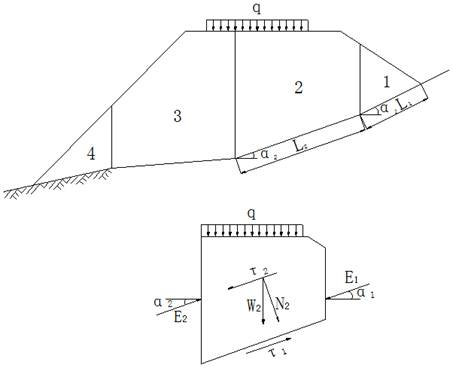Figure 1. Imbalance thrust method illustration.

According to the theory of imbalanced thrust method, it can be concluded that the stability coefficient calculation formula is as follows: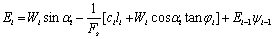(1)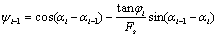(2)

According to formula (1) and (2), after detailed calculating until the remainder of the nth thrust is zero, the stability coefficient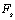is determined.

The related signs to the formula (1) and (2):

Wi—The weight of ith soil slice and the plus vertical load combined;

αi—The inclination of ith soil slice slide face;

ci, φi—The cohesive strength and internal friction angle of ith soil slice undersurface;

li—The length of ith soil slice slide face;

αi--1—The inclination of i-1th soil slice slide face;

Ei-1—The sliding force of ith soil slice transfer to nth soil slice;

The stability of high slope was mainly affected by soil strength parameters of controlling levels. In the engineering practice, the strength parameters of the soil are gotten through experiment according to the actual situation, and the parameters also combine with relevant experience to control.

4. Anchoring Principle and Mechanical Effects Analysis of High Slope

4.1. The Basic Principle of Prestressed Anchor

High slope anchor is a kind of slope reinforcement technology which makes the anchor buried into the slope rock mass through the bolt connection of the system, and makes the unstable rock mass and the stable rock body as a whole. Anchor rod is inserted into the drill hole in advance and the bottom is fixed, then the prestress is exerted to it. The naked part of anchor on the floor with anchor head fixed at its end, one case is that the anchor head is directly reinforced to retaining wall or other structures (such as pile and wall), in order to satisfy the stability of the structure, another case is adopting beam and slab, the lattice or other components, which would transfer the force that the anchor head imposed into rock mass.

There are fundamental difference and outstanding superiority between slope anchorage support and traditional support: anchorage support is not a simple passive force from slope rock mass under loading, but initiative to reinforce the slope rock mass, and it can more effectively control the deformation and prevent sliding of slope rock mass, prestressed anchor rod mechanism is shown in figure 2.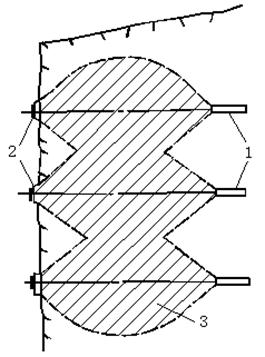1. Anchor roots 2. Anchor head 3. Prestressed rock mass

Figure 2. Prestressed anchor rock strengthen high slope diagram.

In general situation, the intersection angle between bolt axis and the main structural plane or potential damage surface should be large. From figure 3:the rock mass slope anchor settings are shown in figure 3, such as sliding, dumping , rotational failure ,the weathering and denudation of weak zone.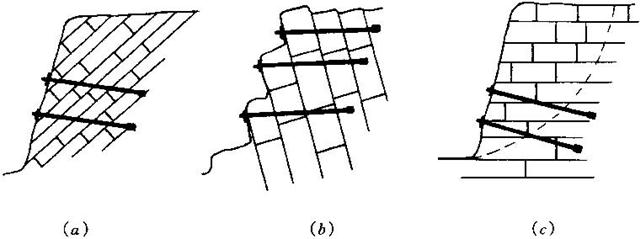Figure 3. Anchor reinforced high slope rock mass stability diagram.

4.2. Bolt Mechanical Effects Analysis of the Slope Reinforcement

The prestressed anchor get through the slope slip plane, the outer is fixed on the slope surface and the inner is fixed within stable rock mass which is under the fracture sliding surface. The prestressed anchor actively changed the stress of the slope rock mass, and the imbalance conditions of the sliding surface force, which can not only improve the integrity of rock mass but also increase the sliding resistance of slip surface, so as to achieve the goal to improve the stability of the slope reinforcement. The prestressed anchor reinforced slope mainly make use of the combination effects of bolt and rock mass, which greatly improve the stability conditions of the slope rock mass. Firstly, due to the effect of prestress, which makes the unstable sliding body under three-dimensionally stressed high confining pressure, which makes the stress state of rock mass and deformation characteristics higher than the uniaxial pressure and low confining pressure conditions. Secondly, the anchorage force of bolt directly change the stress state and sliding stability condition. As is showed in figure 4 and figure 5. The increment of slope sliding resistance increased by the use of prestressed anchor,  Δ P is shown in formula (3):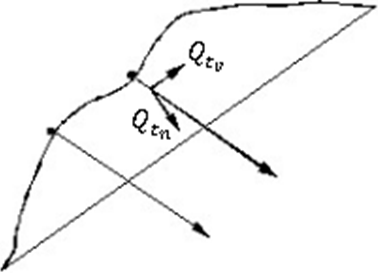Figure 4. Resistance of prestressed anchor.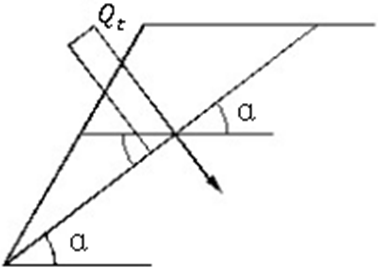Figure 5. Anchoring force system of the sliding surface.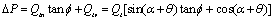(3)

The related signs to the formula (3):

Qt—The design stress of anchor, KN;

Qtn, Qtv—The component force of anchor’s design stress along slide surface’s normal direction and tangent direction,KN;

α—The dip angle of slide face(°);

θ—The angle between anchor and horizontal direction(°);

φ—Internal friction angle of soil slide surface(°).

According to the formula, On the one hand, the anchors directly act on the sliding surface slip and generate resistance; On the other hand, anchor increases the normal stress on the slide surface so as to increase resistance against sliding friction. In short, the main functions of slope anchorage measures is shown as follows:

a. Anchor reinforces and increases the slope rock mass strength, and accordingly improves other mechanical properties of stratum.

b. Anchor produces a compressional stress to the anchored rock mass stratum, or takes reinforcement effect on stratum (non prestressed anchor).

c. When the anchor rod get through the anchored structure, which can make the structure prestressed itself.

d. Through anchor rod, the slope structure and rock mass are interlocked together, and they form a composite structure that makes the rock and soil undertake shearing strength and tensile stress more efficiently.

e. It can effectively confine deformation of rock mass and avoid the strength index’s reduction under the influence of unfavorable factors.

The use of prestressed anchor can play a role of reinforcement in the slope rock mass even loose rock. As a kind of passive mechanical structure, the non prestressed anchor can effectively confine deformation of rock mass, and avoid the strength of reinforced rock mass reducing under the influence of unfavorable factors, so as to achieve the goal of control of slope deformation.

4.3. The Analysis of Anchor in High Slope Stability

Commonly, the problem of slope stability is using slice method to solve. As is shown in figure 6, the stability safety coefficient after setting the prestressed anchor is expressed in formula (4).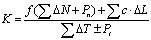(4)

K—-Safety factor of slope;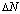——The vertical component of weight act on shear plane, KN;

f——The friction coefficient of shear surface, f=tgφ,φ is the internal friction angle of rock and soil mass;

c——The cohesive force of shear surface, KN;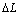——The width of one shear plane, m;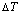——The tangential component of weight act in a shear plane;

Pn——The vertical component of anchoring force, KN. (Notes: The use of non prestressed anchor should not consider the contribution of this index to sliding force.)

Pt——The tangential component of anchoring force, KN;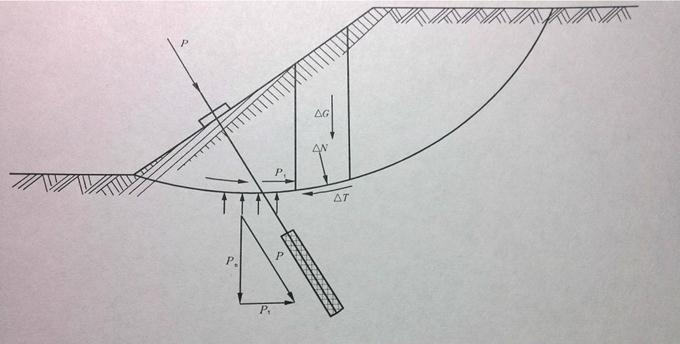Figure 6. Prestressed anchor act in slope stability.

5. Take a High Slope in Chongqing as an Example, Based on the Slide Software to Simulate Anchoring Reinforcement Effect in High Slope

5.1. Parameter Selection

The construction site in combination with geological exploration report, mechanical experiment results and engineering experience, and calculation parameter values are shown in table 1:

Table 1. The geotechnical calculating parameter .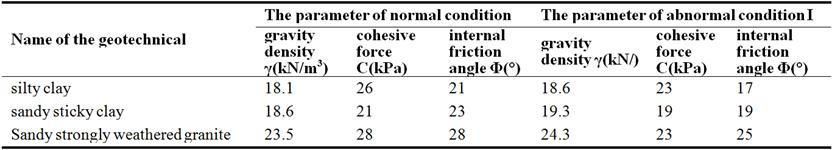5.2. Simulated Condition

The construction site exists rain or continuous rain, the paper should analyze the stability of slope under abnormal conditions I. This slope stability calculation used the simplified Bishop method, according to geotechnical parameter of table 1, the paper set up four kinds of operating mode in the slope stability analysis, as shown in table 2:

Table 2. Simulated condition.

 simulated condition Condition 1 Condition 2 Condition 3 Condition 4 normal condition or abnormal condition I normal condition abnormal condition I normal condition abnormal condition I supporting no no yes yes

According to geological exploration report and combining with engineering experiences, the technical personnel ensure geotechnical parameter based on the analysis of slope deformation mechanism, the paper simulated the process of slope deformation and analysized the deformation trend in the future. The corresponding figures are figure 7, figure 8, figure 9 and figure10.

As figure 7 and figure 8shows, when using natural slope, the most dangerous sliding surfaces are third stage and second stage respectively. Through the calculation, the fracture surface under normal working condition, the slope stability safety coefficient Fs = 0.875< 1.2, and the fracture surface under abnormal condition I, slope stability safety coefficient Fs = 0.850 < 1.1, both did not meet the requirements of specification. And from figure 9, the normal working condition Fs = 1.612 > 1.2, while figure 10 shows abnormal condition I, Fs = 1.404 > 1.1, they meet the specification requirements, which greatly improve the stability of slope.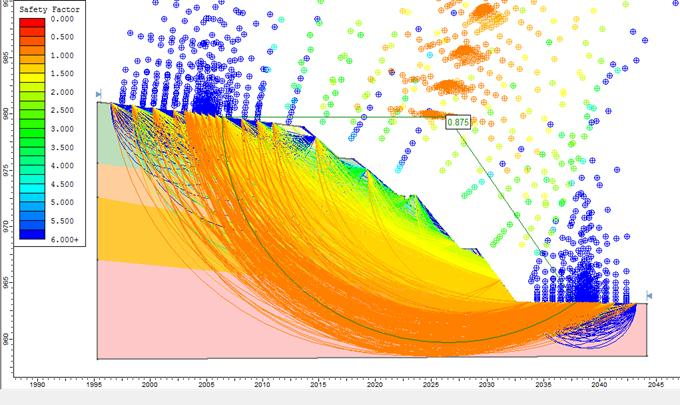Figure 7. Stability calculation of condition 1.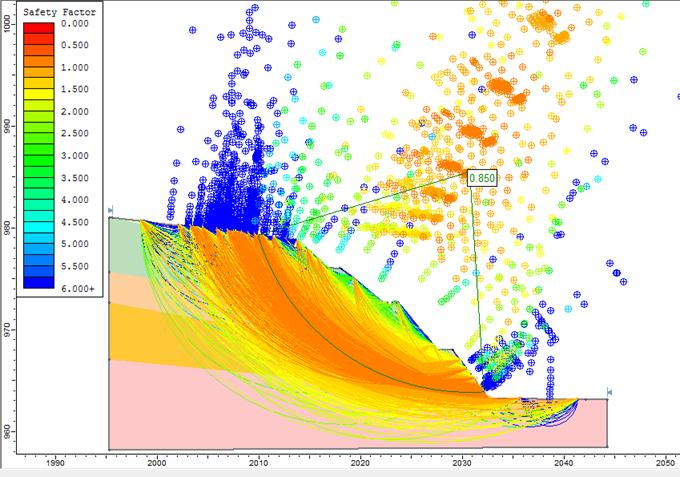Figure 8. Stability calculation of condition 2.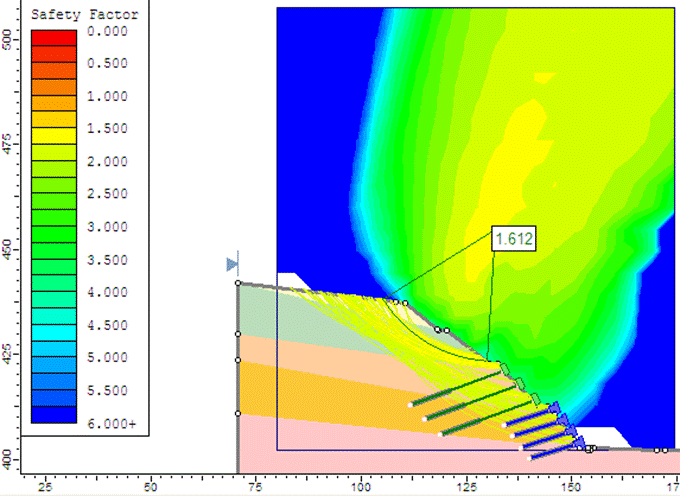Figure 9. Stability calculation of condition 3.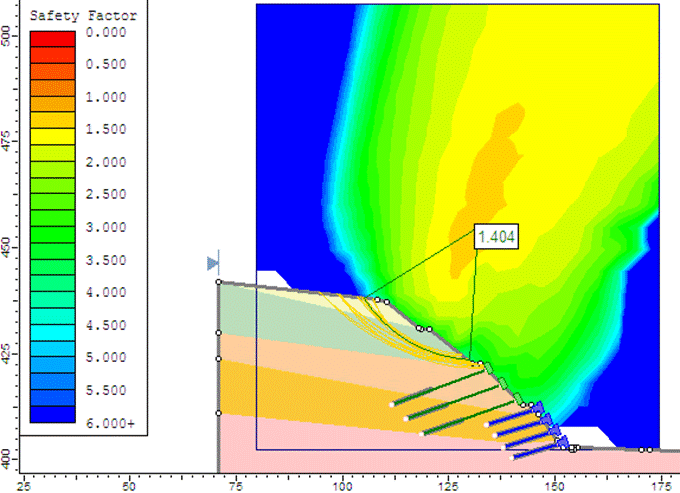Figure 10. Stability calculation of condition 4.

6. Brief Suggestion

From the successful application of anchorage technology in a high slope of Chongqing, two views summed up for similar slopes in future.

1) The soil rock mixture high slope has the diversity of failure mode for it’s characteristics of deformation and material structure, combined with the complexity of the influencing factors, which causes the soil rock mixture slope frequent disease, and the radical cure to the disease is hard. This kind of slope reinforcement design should pay attention to the design principle: namely, considering the fundamental problem of deep sliding deformation, meanwhile accounting for deformation control and processing.

2) Based on anchorage measures, geotechnical anchorage can enhance strength of soil rock mixture body, combined with lattice frame structure, which can play constraint to soil rock mixture body. Anchorage structure has good coordination with geotechnical body, which can make them form a jointworking system, and then try to use their own strength in rock-soil. In short, the geotechnical anchoring technology can be well applied to certain conditions of the soil rock mixture high slope reinforcement.

7. In Conclusion

In highway construction, the soil rock mixture high slope has a variety of deformation and failure mode because of its physical structure characteristics, it has the problem of both shallow water waterlogging and surface soil slumping. Based on its own low strength of soil, which will cause soil mass arc shape sliding problem, there is also a problem controlled by slip problem that the soil rock structure plane glide as a whole. The diversity of deformation failure mode and the complexity of the factors, which will bring frequent disease to the soil rock mixture high slope, and the disease will bring serious impact on the normal construction and the later operation of highway. The soil rock mixture high slope became one of the key problems which puzzled engineering technicians.

On the basis of Slide software, simulation of four kinds of slope stability under different working conditions. According to the minimum stability coefficient of slope under different conditions, which provide a scientific basis for the high slope geological hazard assessment and forecast. High slope stability is influenced by multiple factors, and safety coefficient is low in the heavy rain or continuous rainfall so that the slope is in the condition of instability. But the slope stability of potential sliding surface increased significantly after anchoring, which effectively improve the safety of high slope, and reduce the possibility of safety accidents. Besides, Slide software is convenient and effective in modeling, which simulates high slope stability under different working conditions, it saves time in the actual production and it can make effective evaluation in the stability of high slope.

Acknowledgements

This work has been supported by China Merchants Chongqing Communications Technology Research & Design Institute Co., Ltd., the national science and technology support plan (No.2015BAK09B01).

References

1. Hongyuan Zhou, Zhiyong Zeng. The application of prestressed anchor reinforced frame structure analysis [J]. Journal of railway engineering, 2013, 3: 16-18, 85.
2. Euripides Papamichos, Ioannis Vardoulakis. Rock nail reinforcement of a free surface [J]. International Journal for Numerical and Analytical Methods in Geomechanics, 2012, 36(2).
3. Yaron Toledo, Yehuda Agnon. Three dimensional application of the complementary mild-slope equation [J]. Coastal Engineering, 2011, 58(1).
4. Jörg Schumacher, Maik Boltes, Herwig Zilken et al. Enstrophy amplification events in three-dimensional turbulence [J]. Chaos: An Interdisciplinary Journal of Nonlinear Science, 2008, 18(4).
5. Qiang Yang, Yao Ru Liu, Ying Ru Chen, Wei Yuan Zhou. Deformation reinforcement theory and its application to high arch dams [J]. Science in China Series E: Technological Sciences. 2008 (2).
6. Li Shi, The numerical simulation of prestressed anchorage mechanism and engineering application research [D]. Nanjing: Hohai University, 2007.
7. Dazhao Gao, Juyun Yuan. Soil mechanics and soil science. People's traffic press. 2006.
8. Li Jun, etc., Landslide prestressed anchoring effect and changing laws study [J]. Journal of Jinan University. 2003, 17 (1).
9. Hongbo Zhang, Cataclastic structure rock cutting slope anchoring mechanism analysis and its application research [D]. Shandong, Shandong University, 2002.
10. Griffiths DV,Lane PA.Slope stability analysis by finite elements. Geotechnique. 1999.
11. Spencer E.Method of analysis of the stability of embankments assuming parallel interslice forces. Geotechnique. 1967.
12. Bishop AW.The use of the slip circle in the stability analysis of slopes. Geotechnique. 1955.

 Contents 1. 2. 3. 3.1. 3.2. 4. 4.1. 4.2. 4.3. 5. 5.1. 5.2. 6. 7.
Article ToolsAbstractPDF(3400K)